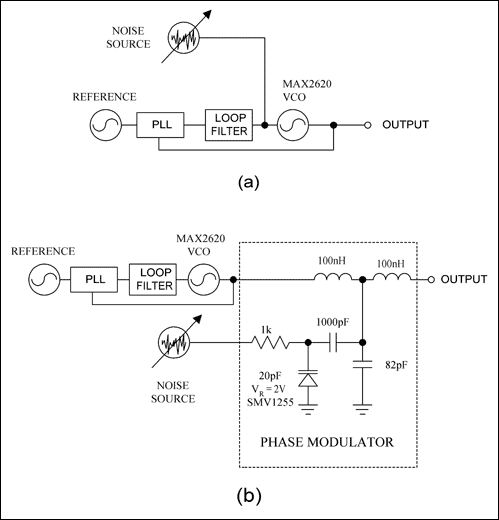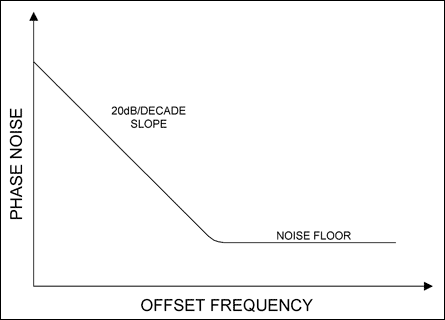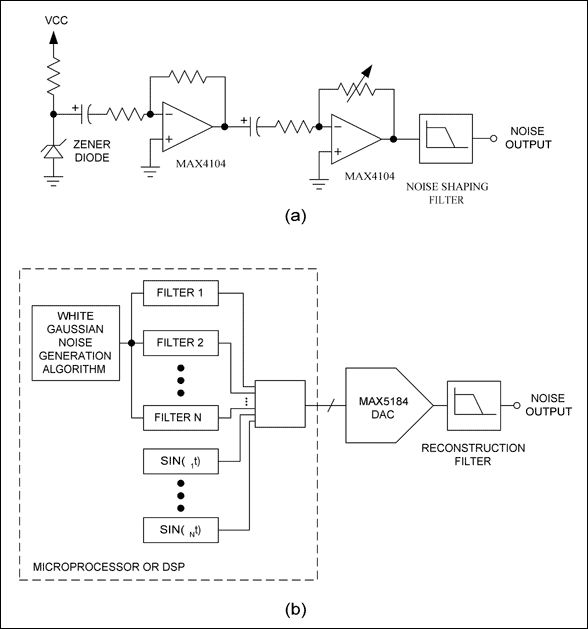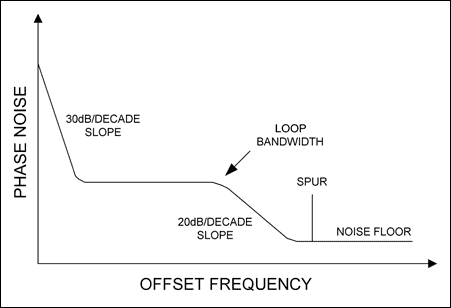# Phase-Noise Profiles Aid System Testing

### Abstract

Noise is present in every system. Phase noise, in particular, is commonly found in oscillators and phase-locked loops can degrade the performance of a system. Phase noise in the oscillator of a wireless communication system degrades the receiver's sensitivity in reciprocal mixing conditions. Phase noise in a telecommunications system causes time jitter in the signal chain. Although engineers usually try to minimize phase noise, sometimes for test purposes they degrade the phase noise intentionally. The deliberate introduction of phase noise aids in testing a system's tolerance for phase noise or jitter. Consequently, signals with an adjustable level of phase noise are useful for testing purposes.

### Introduction

All electronic components contribute to phase noise, but oscillators are generally the dominant source. A voltage-controlled oscillator (VCO), whether free-running or phase-locked, incurs phase noise from noise modulation. It thus follows logically that phase-noise specifications characterize spectral purity. The output of an ideal oscillator, for instance, would be a pure sinusoid represented in the frequency domain as a single-frequency vertical line. In fact, however, oscillators include noise sources that cause the output frequency to deviate from its ideal position, producing a 'skirt' of unwanted frequencies near the carrier.

### Methods for Producing Phase Noise

You can intentionally generate or worsen phase noise in two ways. One way is to directly modulate the oscillator or VCO using a noise source. A VCO (Figure 1a) is phase-locked with a phase-locked loop (PLL), and the loop filter's bandwidth is set lower than the minimum modulating frequency. If, for example, the minimum phase-noise offset frequency of interest is 10Hz (from the carrier), set the PLL loop bandwidth to 1Hz. You inject noise directly into the VCO's frequency-tuning input, where it modulates the VCO to produce phase noise at the output. Then, you can increase the phase-noise level by increasing the input noise-density level.Figure 1. Phase noise is produced by injecting voltage noise directly into the VCO's tuning input (a), or into the varactor diode of a phase modulator (b).

The output phase noise is shaped by the VCO gain (KVCO). Suppose the VCO frequency is ƒo, and is modulated by a noise source of Vn(ƒn) in a bandwidth of 1Hz at frequency ƒn. Using a narrowband approximation for frequency modulation¹, the VCO output is:The first term represents the carrier signal; the second term represents noise power at offset from the carrier. Phase noise is defined as the ratio of noise power at the offset to the carrier power at ƒo:Remember that Vn(ƒn) is the RMS noise voltage in a 1Hz bandwidth at ƒn. The phase-noise profile is the noise-source profile divided by ƒn. Thus, for a white-noise input source with a flat noise-density profile modulating the VCO (Vn(ƒn) = constant), the output phase-noise profile decreases 20dB/decade, as shown in Figure 2. (This assumes that the induced phase noise is much greater than the VCO's intrinsic phase noise.)Figure 2. When a VCO is noise modulated directly at its tuning input, it produces a phase-noise profile with a slope of 20dB per decade.

A second method for producing phase noise uses a phase modulator to modulate the carrier signal at the phase-locked VCO output (Figure 1b). This approach injects noise into the phase modulator, which is a lowpass filter in the LCL configuration². The two inductors are fixed, and the capacitance is made variable using a varactor diode, set to a nominal level of capacitance by the application of reverse bias. Noise voltage across the varactor varies the capacitance which, in turn, varies the phase. Thus, the noise voltage is translated into phase noise. Increasing the noise voltage increases the level of phase noise.

The phase-modulator method does not restrict the PLL loop bandwidth, which can therefore be as wide as necessary to achieve a faster lock time. As another advantage, the phase-noise profile depends not on the VCO gain, but on the phase gain (KPHASE), in units of radians per volt. Phase gain, furthermore, depends on the phase response of the LCL filter and the varactor-diode capacitance characteristics. Thus, the VCO output following the phase modulator is:where Vn(t) is the noise voltage at time, t. The phase-noise term is KPHASEVn(t) = Φ(t). You can calculate phase noise by applying the Fourier transform to VOUT(t), but the result is difficult to solve analytically. As an approximation³, the phase noise is:where SΦ is the spectral density of Φ(t) in rad²/Hz, and Sv(ƒn) is the spectral density of Vn(t) in V²/Hz. The phase-noise profile, therefore, takes the same shape as the modulating noise-density profile. For a white-noise source followed by a 100kHz lowpass filter, the phase-noise profile is the same as the filter's frequency response. In that case, the phase-noise level is constant inside the filter's cut-off frequency, and rolls off outside the -3dB bandwidth (Figure 3). This phase-modulator circuit provides a convenient way to produce a variable phase-noise signal that mimics real-world noisy signal sources such as phase-locked oscillators.Figure 3. This phase-noise profile is produced by the phase modulator in Figure 1b. The shape of the phase-noise profile is the same as the modulating noise-density profile, which is white noise with a 100kHz lowpass filter.

The circuit of Figure 1b works well from 5MHz to 30MHz, and you can easily scale the inductor and capacitor values for operation at other frequencies. Lab experiments show that the circuit can be scaled up to 2GHz or 3GHz. Those frequencies require about 1nH inductance and 1pF capacitance, so the technique is frequency-limited by component availability and PCB parasitics.

A change in the varactor capacitance changes the noise-signal amplitude as well as phase. Amplitude changes are, however, much smaller than the phase changes. The phase changes represent phase noise, and the amplitude changes represent amplitude noise (Figure 4). This modulator produces about 30dB greater phase modulation than amplitude modulation, thereby ensuring that the phase noise predominates.Figure 4. This graph shows phase and amplitude modulation at 10MHz for the Figure 1b circuit. The phase modulation is 30dB greater than the amplitude modulation.

### Generating Noise Voltage

There are many methods for generating noise voltage for the phase-noise modulation. The simplest way is to reverse-bias a zener diode in its avalanche-breakdown region (Figure 5a). The diode's excess shot noise is amplified by both the fixed-gain and the variable-gain amplifiers. The gain of these cascaded amplifiers must be high enough to produce the desired noise-voltage level. The noise output is followed by a filter that shapes the noise according to the phase-noise profile required in Figure 1a or 1b. (An advantage of the circuit in Figure 1b is that the shape of the noise-source profile is the same as the output phase-noise profile.)Figure 5. A zener diode is reverse biased in avalanche-breakdown mode to produce white noise. The white noise is then amplified and filtered to produce a noise profile for phase-noise modulation (a). An advanced noise generator uses a microprocessor to produce a multisegment noise profile, which mimics a more realistic phase-noise profile (b).

The phase-noise profile of an actual oscillator can be complicated. It can roll off at 30dB/decade for low-offset frequencies, become flat inside the loop bandwidth, roll off at 20dB/decade outside the loop bandwidth, and finally, assume a flat noise floor (Figure 6). The phase-noise profile can also have a few sets of reference spurs.Figure 6. In an actual phase-locked oscillator, the phase noise at low-offset frequencies decreases rapidly, with a slope of 30dB/decade. Phase noise is flat inside the loop bandwidth. Outside the loop bandwidth, the phase noise rolls off at 20dB/decade until it reaches the noise floor. Spurs can also appear.

These phase-noise profiles require a more complicated noise-generation circuit, a design like the one shown in Figure 5b. It produces complicated, multisegment noise profiles using a microprocessor or digital signal processor (DSP) and a digital-to-analog converter (DAC). For the phase modulator of Figure 1b, a flat phase-noise region is produced by a white Gaussian-noise voltage followed by a digital filter with flat frequency response in the offset frequency of interest (i.e., a bandpass filter). To produce the required rolloff slope, a white Gaussian noise is followed by a FIR or IIR digital-filter algorithm. For spurs, you can add a sinusoid to the noise voltage. Then, sum all these noise segments together. Still in digital format, the noise voltage is converted to an analog voltage by the DAC, followed by a reconstruction filter.

### Summary

The techniques for producing phase noise are illustrated in Figure 1, and the techniques for producing noise voltage are illustrated in Figure 5. The Figure 1a circuit produces phase noise by modulating the VCO tuning input directly, and the Figure 1b circuit generates phase noise with an external phase modulator. Each technique produces a different phase-noise profile. The direct modulation technique of Figure 1a works at any VCO frequency. For the phase-modulator technique of Figure 1b, the carrier frequency is limited to a few gigahertz by component availability and PC-board parasitics.

References

1. Behzad Razavi, RF Microelectronics. Upper Saddle River, NJ, 1998, pg 223.
2. Enrico Rubiola et. al., "The ±45° Correlation Interferometer as a Means to Measure Phase Noise of Parametric Origin," IEEE Transactions On Instrumentation and Measurement, Vol. 52, No. 1, pp. 182-188.
3. A. L. Lance et. al., "Phase Noise Measurement Systems," ISA Transactions, Vol. 21 No. 4, pp. 37-44.